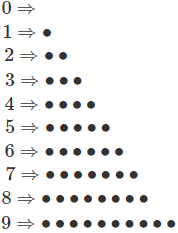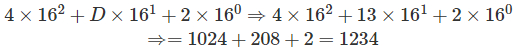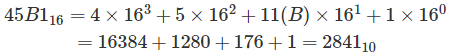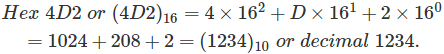The hexadecimal system is a number system with base 16. This number system is greatly used by modern computer system. We already know about the decimal number system, binary number system and octal number system. Like those there is another number system called hexadecimal number system. As the name suggests there are 16 symbols in this number system starting from 0.

Before explaining the number system we should know why this number system came into existence. The natural tendency of human is to use decimal number system because they are familiar with this as the use of 0 is very easy and the operations are user friendly. And the computer systems used binary systems earlier because there are only two states on and off.

But as the dependency on computer grew up and different mathematical programs and different softwares needed to be developed there came the need to develop a number system having base larger than decimal and 16 was chosen because the bits, bytes are multiples of it. Now days this number system is used in HTML and CSS, hexadecimal notations are used in them. This number system was first used around 1956 in Bendix G-15 computer.

Now coming to the representation of hexadecimal number system, in this number system there are 16 basic digits by which all the numbers can be represented, these are 0, 2, 3, 4, 5, 6, 7, 8, 9, A, B, C, D, E, F the first 10 digits are similar to decimal number system but the last 6 digits represent 10, 11, 12, 13, 14 and 15 respectively. Any number in hexadecimal number system can be converted into numbers of other number system very easily, the procedures are given in the next article.Here in the decimal system, we use symbol 1 and 0 side by side that is 10 to represent • • • • • • • • • • + •

That is nine plus one. After that we will have 11 then 12 and so on. That means after nine or 9 we bring back first non – zero digit of symbol that is 1 at left side and repeat all the symbols from 0 to 9 at its right side to represent next ten higher numbers from ten to nineteen (10 – 19). After 19 we put 2 at left and repeat again 0 to 9 to represent next ten higher numbers from twenty to twenty nine (20 – 29).

Decimal number system is very basic number system as ten symbols or digits are used in different combinations to represent all the numbers, this system is said to be of base ten (10). Now think about a number system where you are told to use sixteen symbols instead of 10 symbols. Then what will be your basic construction of the new number system? For that first we have to find out 16 symbols to represent the basic digits of that new number system.

We can create new series of symbols for that, but if we do so it will be very much difficult to remember. That difficulty can be solved if we use commonly used symbols for that purpose. So we can simply use 0 to 9 of decimal system to represents first ten digits 0 to 9 of this new number system.

But for other 6 higher digits there are no symbols available in decimal system so we have to search for them from some commonly used system. We can easily get them from our alphabetical system that means we can use A, B, C, D, E and F as next 6 higher digits (from 10 to 15) in this new number system. The system where total 16 basic digits are used is known as hexadecimal number system.

 A ⇒ • • • • • • • • • • B ⇒ • • • • • • • • • • • C ⇒ • • • • • • • • • • • • D ⇒ • • • • • • • • • • • • • E ⇒ • • • • • • • • • • • • • • F ⇒ • • • • • • • • • • • • • • •

In hexadecimal system we use 16 symbols to represent all numbers. These symbols are 0, 1, 2, 3, 4, 5, 6, 7, 8, 9, A, B, C, D, E and F. After F we use 10 for next higher number 16. Then next increment is 11 which used to represent next natural number 17 and so on.
Hence in hexadecimal system just after F, the first digit becomes 1 and second digit will repeat from 0 to F one by one to represent natural numbers 16 to 31.

That means, 10 ⇒ 16, 11 ⇒ 17, 12 ⇒ 18, 13 ⇒ 19, 14 ⇒ 20, 15 ⇒ 21, 16 ⇒ 22, 17 ⇒ 23, 18 ⇒ 24, 19 ⇒ 25, 1A ⇒ 26, 1B ⇒ 27, 1C ⇒ 28, 1D ⇒ 29, 1E ⇒ 30, 1F ⇒ 31. After this the first digit will increase to 2 and again second digit will repeat from 0 to F one by one to represent natural numbers 32 to 47 and so on.

As we have already stated in the previous articles on number systems that all the number systems are inter related, so as the decimal and hexadecimal numbers. Just as you can convert decimal numbers to binary, or convert decimal numbers to octal numbers, any number in the decimal number system can be converted into the hexadecimal number system.

It will be easy to understand this procedure with a step-by-step example.

Let us first take any decimal number suppose we have taken 7510 and now we want to convert it into hexadecimal number, first we have to divide it by 16.
75/16 = quotient 4, remainder 11
As the quotient is less than 16, we have to stop here and the equivalent hexadecimal number will be
4B8 = 7510
Now we will discuss the method for a slightly bigger number,
Suppose the number is 169310
Now we divide it by 16
1693/16 = quotient = 105, remainder = 13(D)
Now we have to divide the quotient again by 16 and see the result
105/16 = quotient = 6 remainder = 9
As the quotient is less than 16 the calculation part is completed and we can now directly write the result
169310 = 69D16
So the decimal number has been converted into a hexadecimal number.
From above explanation it can be understood that, hexadecimal number is the summation of products of different digit with their respective multipliers. The multipliers are 160, 161, 162, ……..from right hand side or list significant bit (LSB). Let’s have an example 4D2 and this would be expressed asIf we divide decimal 1234 by 16, we will get 77 as quotient and 2 as remainder. Then if we divide decimal 77 by 16, we will get 4 as quotient and 13 or D as remainder. Now if we write side by side from last quotient to first reminder we will get 4D2 which is hexadecimal or hex equivalent of the number 1234.

 For that we divide 1234 by base 16 and we get 77 as the quotient and 2 as the remainder. 16 1234 →2 Divide again 77 by 16 and we get 4 as the quotient and 13 or D as the remainder. 16 77 → D 4

### Video presentation of Decimal to Hexadecimal Conversion

In a similar way any hexadecimal number can be converted into a decimal number. We will look into the process with an example.

But before beginning it should be made clear that before conversion of hexadecimal number all the letters of the number should be taken as their numerical values in decimal number system, i.e. if a digit in hexadecimal number is A then we have to take it as 10, now an example will make the whole procedure clear.

Let us take any hexadecimal number 45B116, we have to convert it into decimal number, so starting from the right most digit we have to start multiplying the digits with ascending power of 16 starting from 0.
So the taken number will be operated asIn this procedure any hexadecimal number can be converted into decimal number.

The value hexadecimal number is determined by multiplying every digit of the hex number by its respective multiplier. We start from LSB or right most digit and multiply it with 160, then come to the next digit at left of LSB and multiply it with 161 and after that we come to the further left digit and multiply 162 with it. We continue this up to MSB or left most bit. The add all this product and finally we get decimal equivalent of hexadecimal number. This one of the easiest process of hexadecimal to decimal conversion.

Think about the hex number 4D2. Here the least significant bit of the number is 2 so we will multiply that with 160 or 1. Then come to the next left digit that is D OR 13 and we will multiply it with 161 or 16. Lastly we will multiply the left most digit or MSB i.e. 4 with 162. Now if we add these three terms, finally we will get the dedcimal equivalent of the said hexadecimal number. This is what Hexadecimal to Decimal Conversion
Hence,Want To Learn Faster? 🎓
Get electrical articles delivered to your inbox every week.
No credit card required—it’s 100% free.